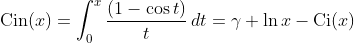Computes the entire cosine intgral.

Namespace:  Meta.Numerics.Functions
Assembly:  Meta.Numerics (in Meta.Numerics.dll) Version: 4.1.4Syntax
```public static double IntegralCin(
double x
)```

#### Parameters

x
Type: SystemDouble
The argument.

#### Return Value

Type: Double
The value of Cin(x).Remarks

The entire cosine integral Cin(x) is related to the conventional cosine integral Ci(x) by:Unlike Ci(x), Cin(x) is regular at the origin, and may therefore be more useful in applications that need the non-divergent part of a cosine integral. In fact, Cin(x) is entire, meaning it has no poles or cuts anywhere in the complex plane. But, unlike Ci(x), Cin(x) does diverge (logarithmicaly) for large x.See Also